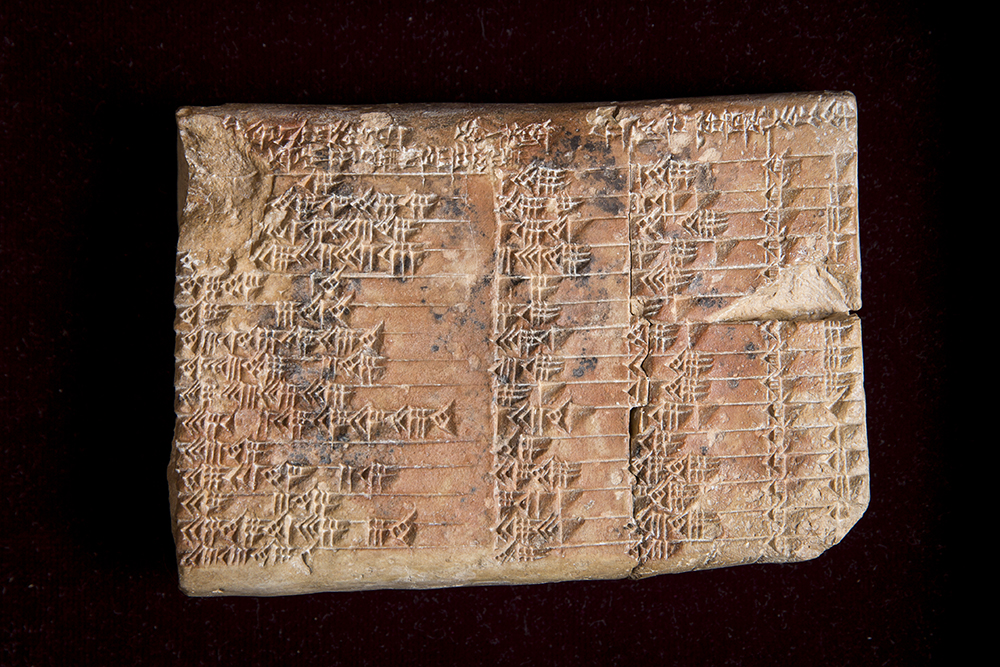# Scientists Decipher Ancient Mathematical Mystery

WORLD

A 3700-year-old Babylonian clay tablet may be the world’s oldest trigonometric table—and in many ways, more accurate than any today. (The Guardian)

What other “firsts” were documented in ancient clay tablets?

Teachers, scroll down for a quick list of key resources in our Teachers Toolkit, including today’s simple MapMaker Interactive map.

Discussion IdeasPlimpton 322 was unearthed in the ancient Babylonian city of Larsa, in what is today Iraq.
• The fascinating new research analyzes Plimpton 322, a 3700-year-old Babylonian clay tablet written in cuneiform. What is cuneiform?
• Cuneiform is a written language made up of different collections of wedge or triangle shapes. Cuneiform was common throughout many cultures of ancient Mesopotamia, including Sumeria, Akkadia, Assyria, and Babylonia.

• The Australian researchers working on Plimpton 322 say it may be the world’s oldest trigonometry table. What is trigonometry?
• Trigonometry is a branch of mathematics dealing with the properties and relationships among angles and sides of triangles. Learn more here.This cuneiform table, Plimpton 322, lists “Pythagorean triples”—numbers that satisfy the Pythagorean equation: a2+b2=c2.Photograph courtesy UNSW/Andrew Kelly
• What trigonometric patterns are found in Plimpton 322?
• Plimpton 322 may be one of the earliest documentations of the famous Pythagorean theorem, possibly the only trigonometry many adults remember. The Pythagorean theorem describes the relation between a right triangle’s hypotenuse (the side opposite the right angle) and the triangle’s other two sides. The Pythagorean equation states that the square of the hypotenuse (c) is equal to the square of the other two sides (a and b): a2+b2=c2.
• Plimpton 322 lists Pythagorean triples, positive integers that satisfy the Pythagorean equation.
• The most famous Pythagorean triple is 3,4,5. Do the math!
• 32+42=52
• 9+16=25
• It checks out.
• The surviving fragment of Plimpton 322 starts with the Pythagorean triple 119, 120, 169. The next triple is 3367, 3456, 4825. This makes sense when you realise that the first triple is almost a square (which is an extreme kind of rectangle), and the next is slightly flatter. In fact, the right-angled triangles are slowly but steadily getting flatter throughout the entire sequence.”

• How were the Pythagorean triples in Plimpton 322 used by Babylonians?
• We’re not entirely sure! As the mathematician in the terrific video above says, scientists have known for decades that the tablet’s “unusual series of numbers proves that the Babylonians knew the Pythagorean theorem a thousand years before Pythagoras was born. But while there is agreement on what the tablet contains, there’s been no agreement on what it was used for.”
• The new research posits that, contrary to modern understanding of trigonometry, regular triangles were not the primary interest of trigonometry in ancient Babylon. Instead, “[g]eometry in ancient Babylon arose from the practical needs of administrators, surveyors, and builders. From their measurements of fields, walls, poles, buildings, gardens, canals, and ziggurats, a metrical understanding of the fundamental types of practical shapes was forged; typically squares, rectangles, trapezoids and right triangles.”

• Why does the mathematician in the video say that the trigonometry in Plimpton 322 may be more accurate than the trig we use today?
• According to the video, “It all comes down to fractions. We count in ‘base 10’ [the decimal system], which only has two exact fractions: .5 and .2 … The Babylonians counted in ‘base 60’ [the sexagesimal system], the same system we use for telling time. This has many more exact fractions … By using this system, the Babylonians were able to make calculations that completely avoided any inexact numbers, thereby avoiding any errors associated with multiplying those numbers.”

• If Babylonian trigonometry was more accurate, why hasn’t it survived?
• Good question. “Perhaps it went out of fashion because the Greek approach using angles is more suitable for astronomical calculations. Perhaps this understanding was lost in 1762 BCE when Larsa [where the tablet was created] was captured by Hammurabi of Babylon. Without evidence, we can only speculate.”

TEACHERS TOOLKIT

Technology for Secondary/College Mathematics: Pythagorean triples

(extra credit!) Historia Mathematica: Plimpton 322 is Babylonian exact sexagesimal trigonometry

## 3 thoughts on “Scientists Decipher Ancient Mathematical Mystery”

1.ourfamiliesblog says:

Reblogged this on ourfamiliesblog.